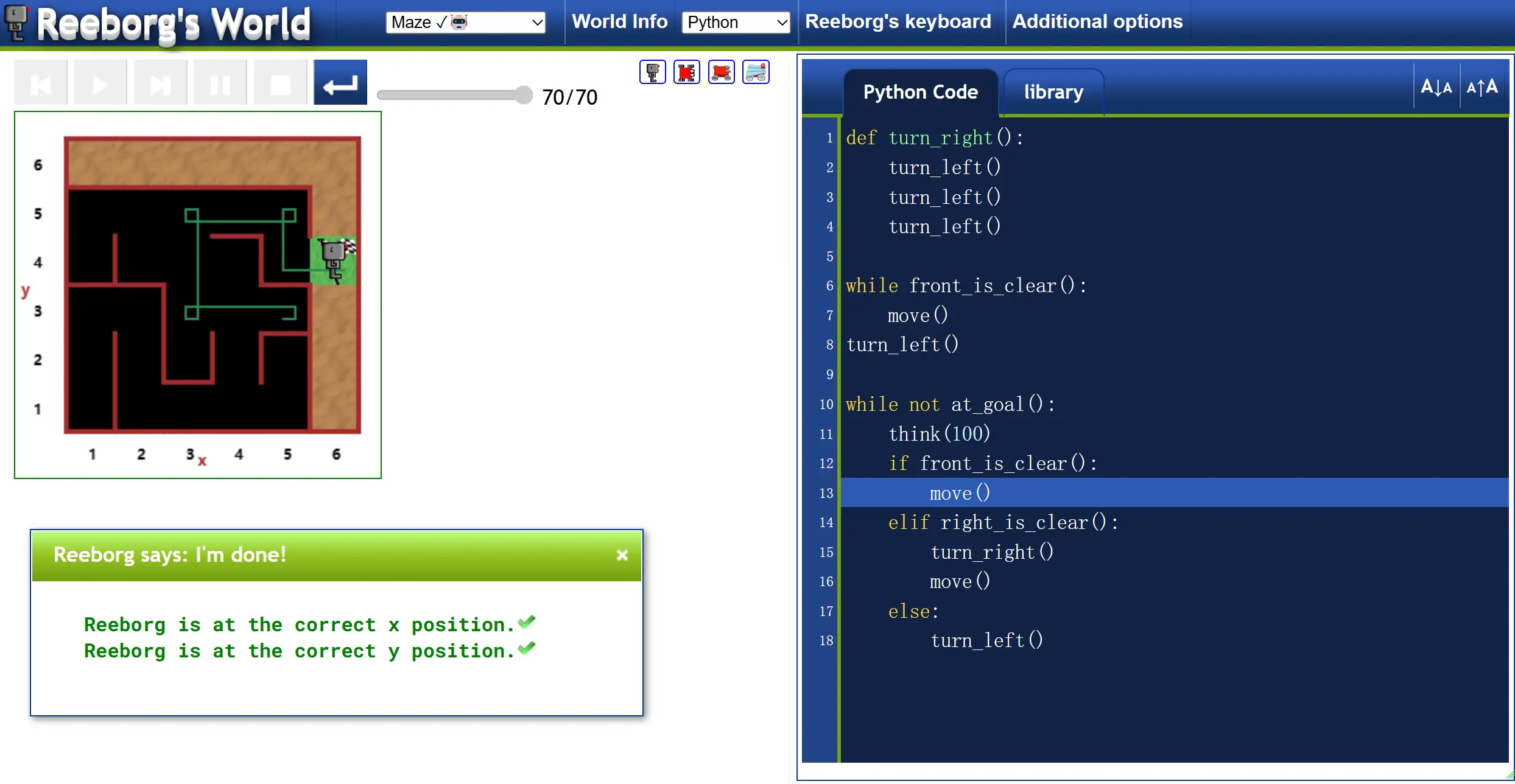### What is Karel

Karel is an educational programming language for beginners, created by Richard E. Pattis in his book Karel The Robot: A Gentle Introduction to the Art of Programming . Pattis used the language in his courses at Stanford University, California. The language is named after Karel Čapek, a Czech writer who introduced the word robot in his play R.U.R.

### What Should You Preview in Advance

Python 基础语法 | 菜鸟教程 (runoob.com)

Python 条件语句 | 菜鸟教程 (runoob.com)

Python 循环语句 | 菜鸟教程 (runoob.com)

Python for 循环语句 | 菜鸟教程 (runoob.com)

Python 函数 | 菜鸟教程 (runoob.com)

Python range() 函数 | 菜鸟教程 (runoob.com)

### Start Up

Reeborg's World

World info 中主要查看当前关卡的一些帮助信息

Reeborg's keyboard 中可以查看有哪些内置命令以及其他指令，同时可以帮助我们快速填充函数

### Hurdle Loop With Fixed Distance (Lv. 1)

# 基础函数
move()
turn_left()

# turn_left()可以让机器人向左转。

def loops():
jump()
jump()
jump()
jump()
jump()
jump()

loops()

# 定义向右转
def turn_right():
turn_left()
turn_left()
turn_left()

# 一个循环
def jump():
move()
turn_left()
move()
turn_right()
move()
turn_right()
move()
turn_left()

# 采用for循环
for step in range(0,6):
jump()

### Hurdle Loop by Using While Loop (Lv. 2)

# 基础函数
move()
turn_left()

# 环境函数
at_goal()

# 额外条件：熟练应用While循环
while loop


# 假设法
while at_goal != True:
jump()

# 反证法
while not at_goal():
jump()

def turn_right():...

def jump():...

while not at_goal():
jump()

### Hurdle Loop by Using While Loop Advanced (Lv. 3)

# 本关所需函数
move()
turn_left()

# 环境函数
front_is_clear()
wall_in_front()
at_goal()

# 额外条件：熟练应用While循环，if条件语句
while loop
if statement

if wall_in_front():
jump()
else:
move()

def turn_right():...

def jump():
turn_left()
move()
turn_right()
move()
turn_right()
move()
turn_left()

while not at_goal():
if wall_in_front():
jump()
else:
move()

### Hurdel Loop With Variable Height (Lv. 4)

def turn_right():...

def jump():
turn_left()
while wall_on_right():
move()
turn_right()
move()
turn_right()
while front_is_clear():
move()
turn_left()

while not at_goal():...

### Maze Escaping (Lv. 5)

def turn_right():...

while front_is_clear():
move()
turn_left()

while not at_goal():
if front_is_clear():
move()
elif right_is_clear():
turn_right()
move()
else:
turn_left()